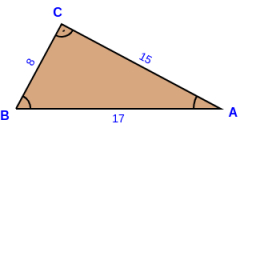# A triangle 4

A triangle has a perimeter of 18a + 6b + 15 and has sides of lengths 5a + 7 and 8a +4b, what is the length of the third side? Write an expression and simplify to find find the solution to the problem.

Result

Z = (Correct answer is: 5 a + 2 b + 8)### Step-by-step explanation:Did you find an error or inaccuracy? Feel free to write us. Thank you!

Tips for related online calculators